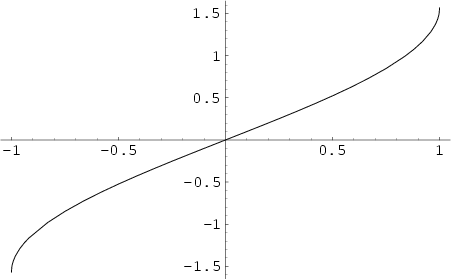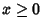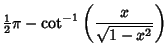## Inverse SineThe function, whereis the Sine and the superscriptdenotes the Inverse Function, not the multiplicative inverse. The inverse sine satisfies(1)

for Positive and Negative, and(2)

for. The inverse sine is given in terms of other inverse trigonometric functions by(3)(4)(5)

for Positive or Negative, and(6)(7)(8)(9)

for.

Abramowitz, M. and Stegun, C. A. (Eds.). Inverse Circular Functions.'' §4.4 in Handbook of Mathematical Functions with Formulas, Graphs, and Mathematical Tables, 9th printing. New York: Dover, pp. 79-83, 1972.